Main Content

# Compute the Histogram of Real and Complex Data

The bin boundaries created by the Histogram block are determined by the data type of the input. The following two models show the differences in the output of the Histogram block based on the data type of the input.

### Real Input Data

When the input data is real, the bin boundaries are cast into the data type of the input.

Open the model.

```modelRealData = 'ex_realData_hist'; open_system(modelRealData) ```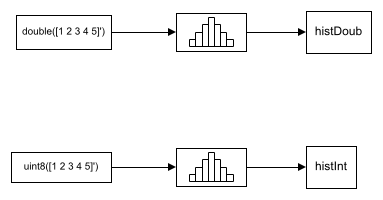Run the model.

```sim(modelRealData) ```
```Warning: Reported in '<a href="matlab:open_and_hilite_hyperlink ('ex_realData_hist/Histogram1','error')">ex_realData_hist/Histogram1</a>': The bin width resulting from the specified parameters is less than the precision of the input data type. This might cause unexpected results. Since bin width is calculated by ((upper limit - lower limit)/number of bins), you could increase upper limit or decrease lower limit or number of bins. ```

The block produces two histogram outputs.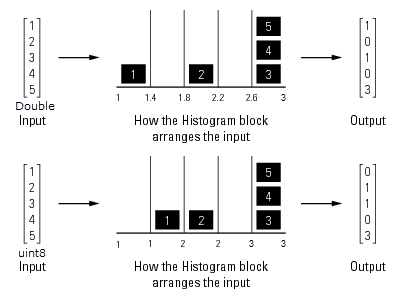The output of the Histogram block differs based on the data type of the input. A warning occurs in the second histogram block, where the bin boundaries are uint8([1 1.4 1.8 2.2 2.6 3.0]) = [1 1 2 2 3 3]. The width of the first and third bins are 0, and the precision of the data is 1. The block expects the width of each bin to be at least equal to 1.

To resolve this warning, increase the upper limit of second Histogram block to 7 and decrease the number of bins to 2. The bin width becomes ((7-1)/2) = 3. With the integer input, the new bin boundaries are uint8[1 4 7] = [1 4 7]. The bins are spread out more evenly.

```set_param('ex_realData_hist/Histogram1','umax','7','nbins','2'); ```

Simulate the model. The warning no longer appears and the bins spread out more evenly.

```sim(modelRealData) ```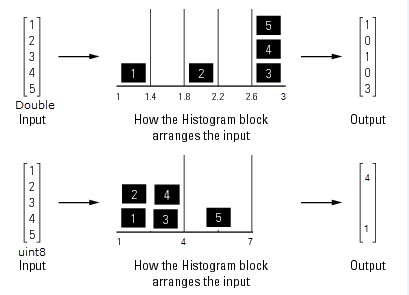### Complex Input Data

When the input data is complex:

• Bin boundaries for double-precision inputs are cast into the double data type. All complex, double-precision input values are placed in the bins according to their magnitude, which is the square root of the sum of the squares of the real and imaginary parts.

• Bin boundaries for integer inputs are cast into the data type double and squared. All complex, integer input values are placed in bins according to their magnitude-squared value.

Open the model.

```modelComplexData = 'ex_complexData_hist'; open_system(modelComplexData) ```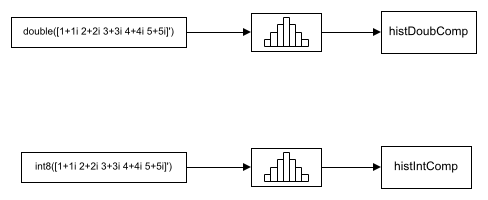Run the model.

```sim(modelComplexData) ```
```Warning: Reported in '<a href="matlab:open_and_hilite_hyperlink ('ex_complexData_hist/Histogram1','error')">ex_complexData_hist/Histogram1</a>': The bin width resulting from the specified parameters is less than the precision of the input data type. This might cause unexpected results. Since bin width is calculated by ((upper limit - lower limit)/number of bins), you could increase upper limit or decrease lower limit or number of bins. ```

The model produces two histogram outputs.The top figure shows the histogram for the double-precision input, and the bottom figure shows the histogram for the integer input. The double-precision inputs are normalized for comparison, whereas the integer inputs are placed using their magnitude-squared value. A warning occurs in the second histogram block, where the bin boundaries are [1 (1.4)² (1.8)² (2.2)² (2.6)² (3.0)²]. The precision of the data is at least 6, and the width of the bins is less than 2.

To resolve this warning, increase the upper limit of second Histogram block to 10. With the new upper limit, the bin boundaries are [1 (2.8)² (4.6)² (6.4)² (8.2)² 10²] = [1 7.84 21.16 40.96 67.24 100].

```set_param('ex_complexData_hist/Histogram1','umax','10'); ```

Simulate the model. The warning no longer appears and the bins in the second Histogram block are spread out more evenly.

```sim(modelComplexData) ```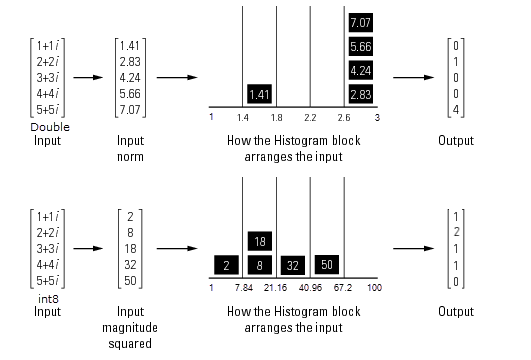Save and close the models.

```save_system(modelRealData); save_system(modelComplexData) close_system(modelRealData); close_system(modelComplexData); ```

Watch now